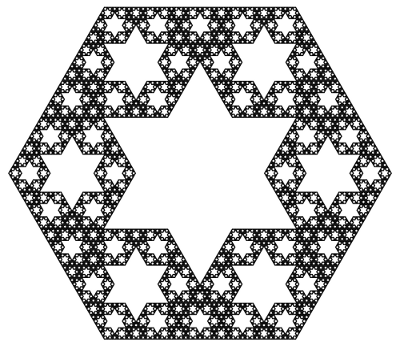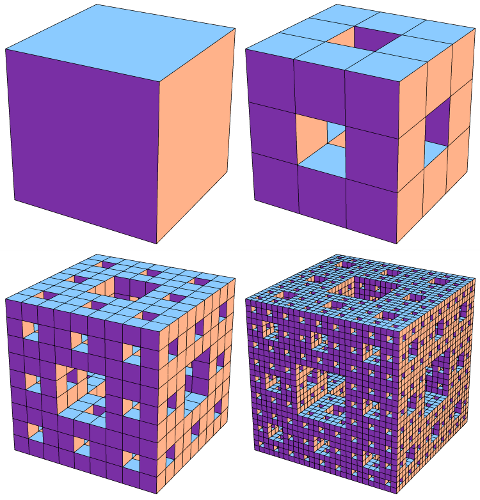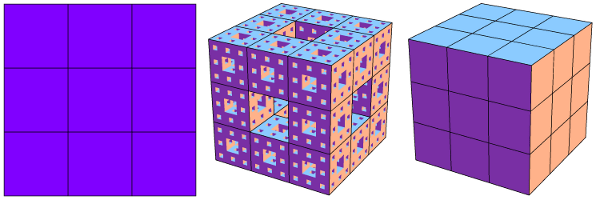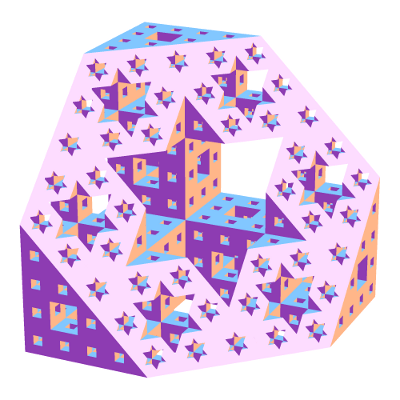# Seeing Stars

Let us now take a closer look at the hex-fractal we sliced last week. Chopping a level 0, 1, 2, and 3 Menger sponge through our slanted plane gives the following:

This suggests an iterative recipe to generate the hex-fractal. Any time we see a hexagon, chop it into six smaller hexagons and six triangles as illustrated below. Similarly, any time we see a triangle, chop it into a hexagon and three triangles like this:A recipe for constructing the hex-fractal: replace each hexagon with 6 hexagons and 6 triangles, and replace each triangle with 1 hexagon and 3 triangles.

In the limit, each triangle and hexagon in the above image becomes a hex-fractal or a tri-fractal, respectively. The final hex-fractal looks something like this (click for larger image):Now we are in a position to answer last week’s question: how can we compute the Hausdorff dimension of the hex-fractal? Let d be its dimension. Like last week, our computation will proceed by trying to compute the “d-dimensional volume” of our shape. So, start with a “large” hex-fractal and tri-fractal, each of side-length 1, and let their d-dimensional volumes be h and t respectively.

Break these into “small” hex-fractals and tri-fractals of side-length 1/3, so these have volumes $$h/3^d$$ and $$t/3^d$$ respectively (this is how “d-dimenional stuff” scales). Since $$\begin{gather*}(\text{large hex}) = 6(\text{small hex})+6(\text{small tri}) \quad \text{and}\\ (\text{large tri}) = (\text{small hex})+3(\text{small tri}),\end{gather*}$$ we find that $$h=6h/3^d + 6t/3^d$$ and $$t=h/3^d+3t/3^d$$. Surprisingly, this is enough information to solve for the value of $$3^d$$. We find $$3^d = \frac{1}{2}(9+\sqrt{33})$$, so $$d=\log_3\left(\frac{9+\sqrt{33}}{2}\right) = 1.8184\ldots,$$ as claimed last week.

As a final thought, why did we choose to slice the Menger sponge on this plane? Why not any of the (infinitely many) others? Even if we only look at planes parallel to our chosen plane, a mesmerizing pattern emerges:

It takes a bit more work to turn the above computation of the hex-fractal’s dimension into a full proof, but there are a few ways to do it. Possible methods include mass distributions or similarity graphs.

This diagonal slice through the Menger sponge has been proposed as an exhibit at the Museum of Math. Sebastien Perez Duarte seems to have been the first to slice a Menger sponge in this way (see his rendering), and his animated cross section inspired my animation above.

### Notes

1. We’re assuming that the hex-fractal and tri-fractal have the same Hausdorff dimension. This is true, and it follows from the fact that a scaled version of each lives inside the other. []
2. There are actually two solutions, but the fact that h and t are both positive rules one out. []
3. Proposition 4.9 in: Kenneth Falconer. Fractal Geometry: Mathematical Foundations and Applications. John Wiley & Sons: New York, 1990. []
4. Section 6.6 in: Gerald Edgar. Measure, Topology, and Fractal Geometry (Second Edition). Springer: 2008. []

# A Slice of Interdimensional Sponge Cake

The Menger sponge is a delightfully pathological shape to soak up. Start with a cube of side-length 1, and “hollow it out” by slicing it into 27 sub-cubes (like a Rubik’s Cube) and removing the 7 central sub-cubes as illustrated below. Call this the level 1 sponge. To make the 2nd level, perform the same “hollowing out” operation on each of the 20 cubes in level 1. Keep going to make levels 3, 4, and so on. The end result, i.e., level $$\infty$$, is a fractal known as the Menger Sponge. It has a crazy network of tunnels and passageways running through it:Stages in the construction of the Menger sponge: Levels 0, 1, 2, and 3. Click for larger image.

What is its volume? We made the Menger Sponge by cutting out smaller and smaller chunks from the original unit cube, so how much volume did we leave behind? Each “hollowing out” step reduces the volume by a factor of 20/27, so level k has volume $$(20/27)^k$$ and the final Menger Sponge has volume 0. We removed all the volume!

Since the Menger Sponge doesn’t have enough “3D stuff” to be considered a truly 3-dimensional shape, maybe it behaves more like a 2-dimensional surface? This isn’t quite right either. The Menger Sponge has “too much 2D stuff”: its surface area is infinite. In fact, this shape falls squarely (hah!) in the middle: it has dimension 2.7268….

Wait, what does it mean to have non-integer dimension? One interpretation, formalized in the notion of Hausdorff dimension, is to look at how the “stuff” it is made from behaves under scaling. If we take a square (which is made of “2-dimensional stuff”) and scale it by a factor of 1/3, its area changes by a factor of $$(1/3)^2$$. Similarly, scaling a cube (“3D stuff”) by 1/3 scales its volume by $$(1/3)^3$$. In general, scaling “d-dimensional stuff” by a factor of 1/3 scales its “d-dimensional volume” by $$(1/3)^d$$.How different dimensional "stuff" behaves when scaled by a factor of 1/3. Left: A 2D plane is split into 9=3^2 smaller copies. Right: A 3D cube is split into 27=3^3 copies. Middle: A Menger sponge is split into 20 smaller copies.

As shown in this image, a Menger Sponge can be chopped into 20 smaller Menger Sponges, each scaled by 1/3. So if the Menger Sponge is made of m-dimensional stuff, its m-dimensional volume scales by 1/20 when the shape is scaled by 1/3, so we should have $$(1/3)^m=1/20$$, i.e., $$m=\log_3(20) = 2.7268\ldots$$.

As one more example of the quirkiness that can be squeezed out of the Menger Sponge, what happens when we slice this shape along one of its primary diagonal planes? The Menger Sponge’s crazy network of tunnels creates a stellar array of 6-pointed stars cut out of a regular hexagon:Slicing a Menger sponge along a primary diagonal plane produces a dazzling fractal (living in the slicing plane) made from 6-pointed stars.

What is the Hausdorff dimension of this hexagonal fractal? It turns out to be $$\log_3\left(\tfrac{9+\sqrt{33}}{2}\right) = 1.8184\ldots.$$ To find out where this ridiculous number comes from, you’ll have to come back next week!

### Notes

1. This is a technical term, of course. []
2. The surface area of level k can be computed to be $$2\cdot(20/9)^k+4\cdot(8/9)^k$$, and this approaches infinity as k grows. []
3. Specifically, slice it along the perpendicular-bisecting plane of one of the cube’s diagonals []